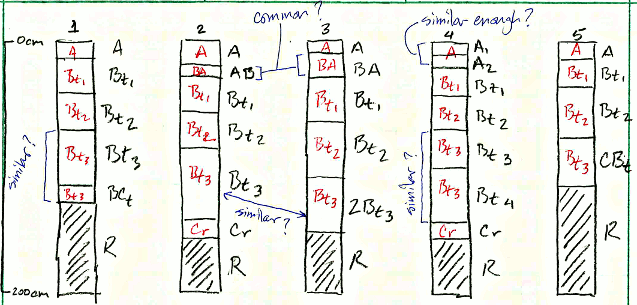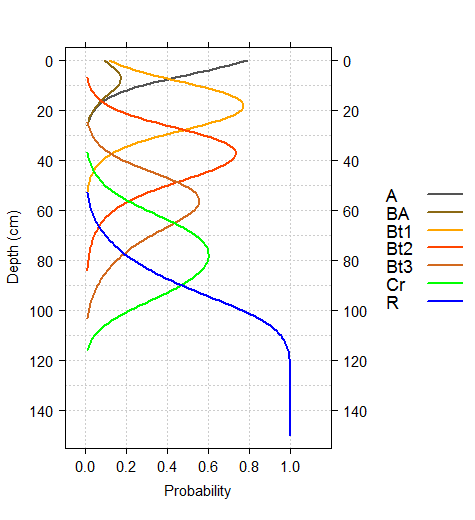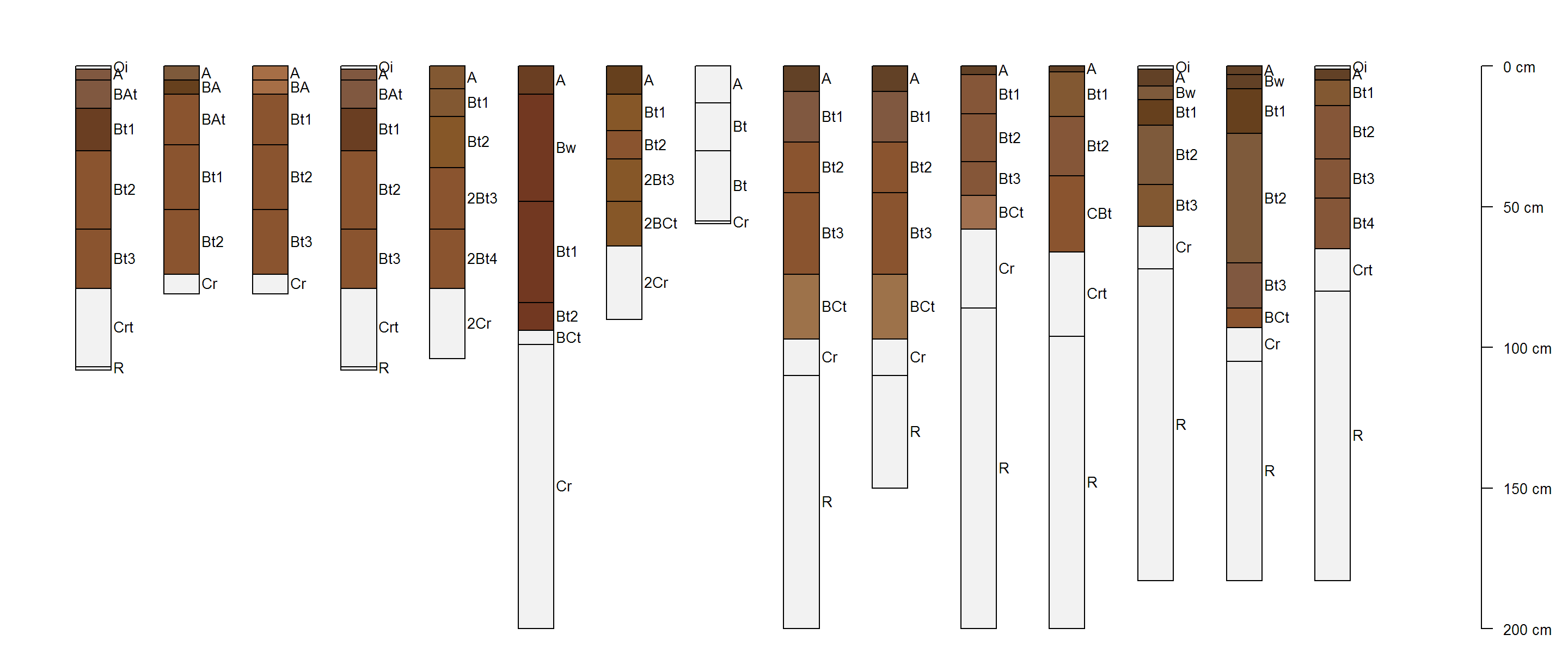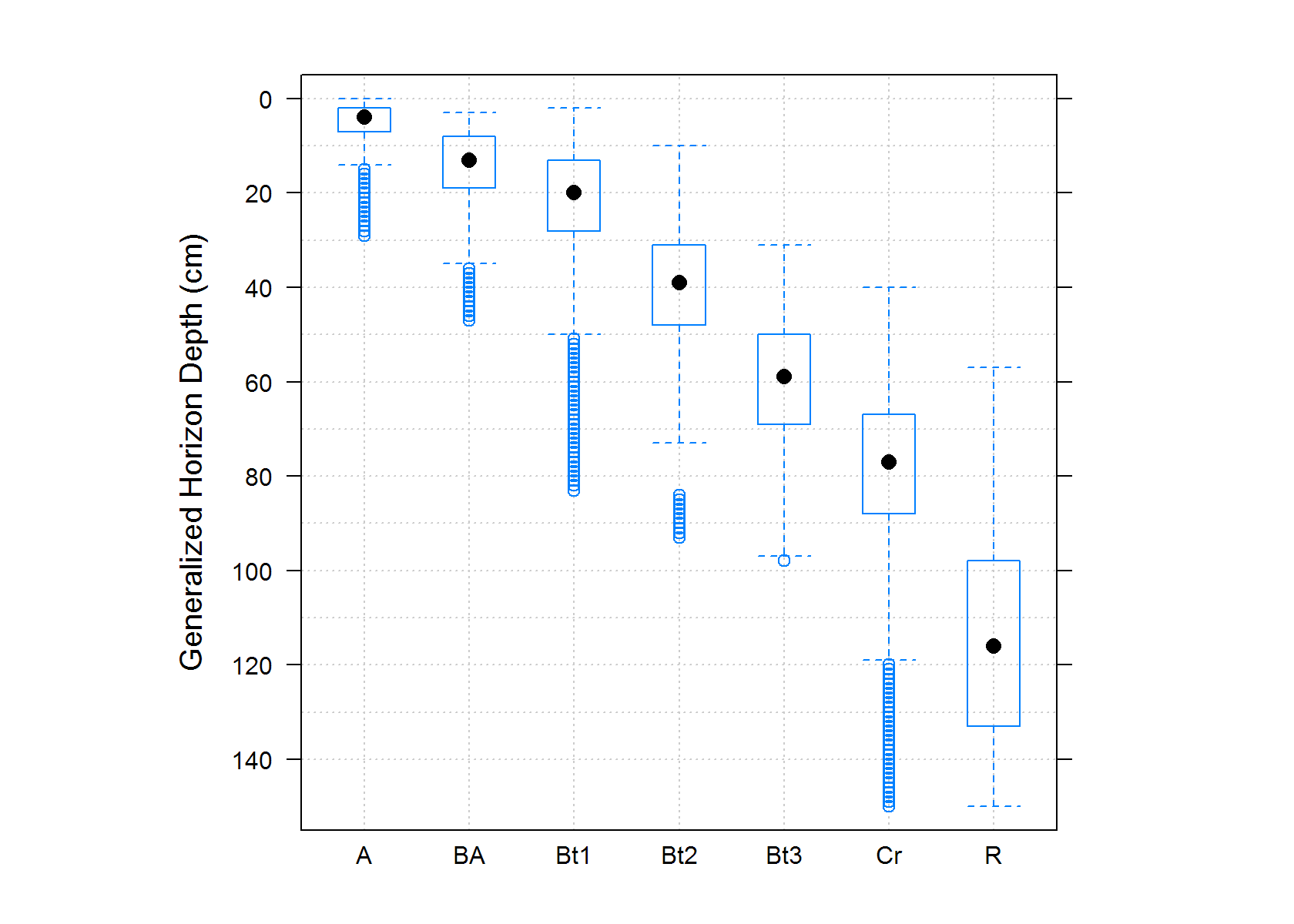# Estimation of Most-Likely Horizon Depths

D.E. Beaudette, J.M. Skovlin
2016-08-17
This document is based on aqp version 1.9.11 and soilDB version 1.8-1.

# Introduction

## Review

Consider this situation: you have a collection of pedons that have been correlated to a named soil series (or component) and would like to objectively compute a range in characteristics (“low-rv-high” values) and horizon depths. As with most collections of pedon data, there may be considerable variation in description style and horizons used, horizon depths, and number of horizons described:alt text

In this scenario, there are several obvious “micro-correlation” decisions that need to be made before horizons can be grouped for aggregation. For example, what horizonation prototype scheme (e.g., A-Bt1-Bt2-Bt3-Cr-R) best conveys the concept of this soil series or soil component? Does it make sense to group [Bt3, Bt4, BCt, CBt] horizons for aggregation? Or what about grouping [Bt3, 2Bt3] horizons? Do [BA, AB] horizons occur frequently enough to be included in the horizonation prototype?

Based on your knowledge of the area, pedon 2 might be a good “typical” pedon to use in developing a horizonation prototype. After careful review of the data and consultation with your crew, a new set of labels are assigned to each horizon (red labels in figure above) that define groups over which soil properties will be aggregated. These new labels define functionally similar groups that may span multiple genetic horizons.

## Probabalistic Representation of Horizon Depths

Once you have assigned generalized horizon labels (GHL) to your pedons it is possible to generate a set of “most-likely” horizon depths for each GHL. An evaluation GHL probability along 1-cm depth slices results in a set of probability depth functions; the points at which these curves cross are good estimates of “most-likely” horizon depths. For example, in the figure below approximate horizon depths of 8, 28, 50, and 90cm can be readily estimated from the probability depth functions. It is also possible to visually determine when less common horizons (such as the BA horizon in the figure below) may not be common enough to include in a representative profile.alt text

# Setup R Envionment

If you have never used the aqp or soildb packages before, you will likely need to install them. This only needs to be done once.

# stable version from CRAN + dependencies
install.packages('aqp', dep=TRUE)
install.packages('soilDB', dep=TRUE)
install.packages('aqp', repos="http://R-Forge.R-project.org")
install.packages('soilDB', repos="http://R-Forge.R-project.org")

Once you have all of the R packages on which this document depends, it is a good idea to load them. R packages must be installed anytime you change versions of R (e.g., after an upgrade) and loaded anytime you want to access functions from within those packages.

library(aqp)
library(soilDB)
library(latticeExtra)
library(plyr)
library(rms)

# Sample Data

While the methods outlined in this document can be applied to any collection of pedons, it is convenient to work with a standardized set of data. You can follow along with the analysis by copying code from the following blocks and running it in your R session. The sample data used in this document is based on 15 soil profiles that have been correlated to the Loafercreek soil series from the Sierra Nevada Foothill Region of California. Note that the internal structure of the loafercreek data is identical to the structure returned by fetchNASIS() from the soilDB package. All horizon-level values are pulled from the pedon horizon table of the pedons being analyzed.

# load sample data from the soilDB package
data(loafercreek, package = 'soilDB')
pedons <- loafercreek
# plot the first 15 profiles
par(mar=c(0,0,0,0))
plot(pedons[1:15, ], name='hzname', print.id=FALSE, cex.names=0.8, axis.line.offset=-4)15 pedons correlated to the Loafercreek soil series.

The following code block demonstrates how to pull data in using the fetchNASIS() function from the soilDB package.

# first load the desired data set within NASIS into your NASIS selected set
# then load data from the NASIS selected set into R
pedons <- fetchNASIS()
# optionally subset the data by taxon name - enter your taxon name
pedons <- pedons[grep(pattern='ENTER_YOUR_TAXON_NAME', pedons$taxonname, ignore.case=TRUE), ] # Methods # apply GHL rules n <- c("A", "BA", "Bt1", "Bt2", "Bt3", "Cr", "R") p <- c("^A$|Ad|Ap|^ABt$", "AB$|BA$|Bw", "Bt1$|^Bt$", "^Bt2$", "^Bt3|^Bt4|CBt$|BCt$|2Bt|2CB$|^C$", "Cr", "R")
pedons$genhz <- generalize.hz(pedons$hzname, n, p)

# remove non-matching generalized horizon names
pedons$genhz[pedons$genhz == "not-used"] <- NA
pedons$genhz <- factor(pedons$genhz)

# keep track of generalized horizon names for later
hz.names <- levels(pedons$genhz) # slice GHL into 1cm intervals: no aggregation max.depth <- 150 slice.resolution <- 1 slice.vect <- seq(from = 0, to = max.depth, by = slice.resolution) s <- slice(pedons, slice.vect ~ genhz) # convert GHL to factor s$genhz <- factor(s$genhz, levels = hz.names) # plot depth-ranges of generalized horizon slices bwplot(hzdept ~ genhz, data = horizons(s), ylim = c(155, -5), ylab = "Generalized Horizon Depth (cm)", scales = list(y = list(tick.number = 10)), asp = 1, panel = function(...) { panel.abline(h = seq(0, 140, by = 10), v = 1:length(hz.names), col = grey(0.8), lty = 3) panel.bwplot(...) })## TODO: abstract most of this into a convenience function # proportional-odds logistics regression: fits well, ignore standard errors using sliced data # properly weights observations... but creates optimistic SE rcs required when we include depths > # 100 cm... should we use penalized PO-LR? see pentrace() dd <- datadist(horizons(s)) options(datadist = "dd") (l.genhz <- orm(genhz ~ rcs(hzdept), data = horizons(s), x = TRUE, y = TRUE)) ## ## Logistic (Proportional Odds) Ordinal Regression Model ## ## orm(formula = genhz ~ rcs(hzdept), data = horizons(s), x = TRUE, ## y = TRUE) ## Frequencies of Responses ## ## A BA Bt1 Bt2 Bt3 Cr R ## 440 182 1203 1276 1054 1117 1651 ## ## Frequencies of Missing Values Due to Each Variable ## genhz hzdept ## 2590 0 ## ## ## Model Likelihood Discrimination Rank Discrim. ## Ratio Test Indexes Indexes ## Obs 6923 LR chi2 12887.35 R2 0.868 rho 0.929 ## Unique Y 7 d.f. 4 g 5.722 ## Median Y 5 Pr(> chi2) <0.0001 gr 305.564 ## max |deriv| 5e-04 Score chi2 11732.53 |Pr(Y>=median)-0.5| 0.409 ## Pr(> chi2) <0.0001 ## ## Coef S.E. Wald Z Pr(>|Z|) ## y>=BA -1.2790 0.0902 -14.17 <0.0001 ## y>=Bt1 -2.0662 0.0948 -21.80 <0.0001 ## y>=Bt2 -5.9137 0.1648 -35.88 <0.0001 ## y>=Bt3 -9.0995 0.1993 -45.67 <0.0001 ## y>=Cr -11.4069 0.2085 -54.72 <0.0001 ## y>=R -13.9939 0.2201 -63.59 <0.0001 ## hzdept 0.2112 0.0061 34.80 <0.0001 ## hzdept' -0.2966 0.0295 -10.06 <0.0001 ## hzdept'' 0.6433 0.0897 7.17 <0.0001 ## hzdept''' -0.5940 0.1348 -4.41 <0.0001 # predict along same depths: columns are the class-wise probability fitted.ind --> return all # probability estimates p <- data.frame(predict(l.genhz, data.frame(hzdept = slice.vect), type = "fitted.ind")) # re-name, rms model output give funky names names(p) <- hz.names # add depths p$top <- slice.vect

p.ml <- get.ml.hz(p, hz.names)

p.ml
##    hz top bottom confidence pseudo.brier
## 1   A   0      9         54   0.26535710
## 2 Bt1   9     30         62   0.22608888
## 3 Bt2  30     50         58   0.27730934
## 4 Bt3  50     68         48   0.39411511
## 5  Cr  68     91         52   0.35529430
## 6   R  91    151         85   0.06798828